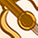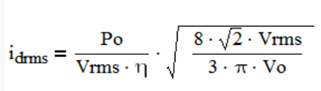# Power Management ICs Forum DiscussionsLevel 3
Level 3# RMS current of MOSFET for CCM PFC use ICE3PCS03G

Hello IFXer,

Do you know how to calculate the the RMS current of MOSFET for CCM PFC?

1 SolutionModeratorModerator# Re: RMS current of MOSFET for CCM PFC use ICE3PCS03G

Hi,
Thanks for posting at Infineon Community.
Please refer to page No. 12 in the below reference application note.
https://www.infineon.com/dgdl/InfineonApplicationNote_PFCCCMBoostConverterDesignGuide-AN-v02_00-EN.p...

Steps to Derive FET RMS Current.
1. Calculate Duty Cycle for PFC CCM Mode (Below shown final result)

D = 1-VIN/VO

2. Calculate Inductor RMS Current (Below shown final result)

ILRMS = (PO/VRMS X ƞ)

3. RMS current during one switching period can be calculated using below formula

IQRMS = ILRMS X √D

Since all quantities are a function of wt due to sin input. The above equation can be written as below.

IQRMS (ωt) = ILRMS (ωt) X √D(ωt)

By substituting, step 1 and 2 into 3 below equation can be derived

IQRMS (ωt) = ((√2 X PO |sinωt|)/(VRMS X ƞ) X (√1-(√2 X VRMS X |sinωt|)/VO)

4. By Integrating mean square value of the above equation we can get the resultant FET RMS equation as belowNote: PF Considered as 1

Thanks,

SPCH.ModeratorModerator# Re: RMS current of MOSFET for CCM PFC use ICE3PCS03G

Hi,
Thanks for posting at Infineon Community.
Please refer to page No. 12 in the below reference application note.
https://www.infineon.com/dgdl/InfineonApplicationNote_PFCCCMBoostConverterDesignGuide-AN-v02_00-EN.p...

Steps to Derive FET RMS Current.
1. Calculate Duty Cycle for PFC CCM Mode (Below shown final result)

D = 1-VIN/VO

2. Calculate Inductor RMS Current (Below shown final result)

ILRMS = (PO/VRMS X ƞ)

3. RMS current during one switching period can be calculated using below formula

IQRMS = ILRMS X √D

Since all quantities are a function of wt due to sin input. The above equation can be written as below.

IQRMS (ωt) = ILRMS (ωt) X √D(ωt)

By substituting, step 1 and 2 into 3 below equation can be derived

IQRMS (ωt) = ((√2 X PO |sinωt|)/(VRMS X ƞ) X (√1-(√2 X VRMS X |sinωt|)/VO)

4. By Integrating mean square value of the above equation we can get the resultant FET RMS equation as belowNote: PF Considered as 1

Thanks,

SPCH.